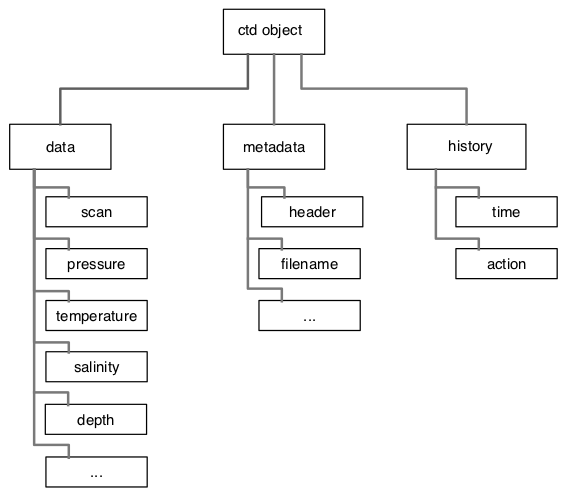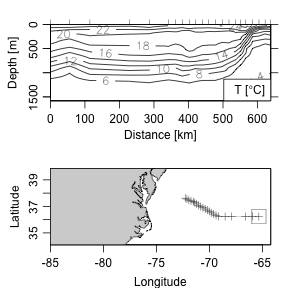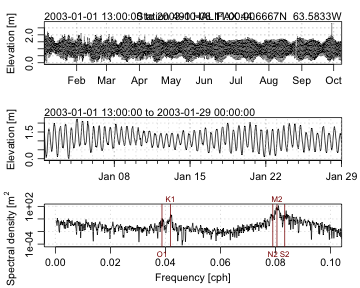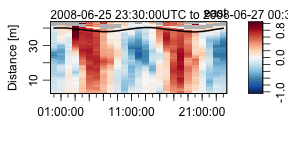# 1. Introduction to Oce

#### 2020-02-21

Abstract. The oce package makes it easy to read, summarize and plot data from a variety of Oceanographic instruments, isolating the researcher from the quirky data formats that are common in this field. It also provides functions for working with basic seawater properties such as the equation of state, and with derived quantities such as the buoyancy frequency. Although simple enough to be used in a teaching context, oce is powerful enough for a research setting. These things are illustrated here in a general context; see also the vignettes on using data-quality flags and processing data from acoustic Doppler profilers.

# 1 Introduction

Oceanographers must deal with measurements made by a wide variety of instruments, a task that is complicated by a tendency of instrument manufacturers to invent new data formats. Although manufacturers often provide software for scanning data files and producing overview plots, this software is of limited use to researchers who work with several instrument types at the same time, and who need to move beyond engineering plots to scientific plots and statistical analysis.

# 2 Architecture of oce objectsFigure 1. Basic layout of a CTD object. All oce objects contain slots named data, metadata, and processingLog, with the contents depending on the type of data.

The need to scan diverse data files was one motivation for the creation of oce, but an equal goal was to make it easy to work with the data once they are in the system. This was accomplished partly by the provision of specialized and generic (overloaded) functions to work with the data, and partly by providing accessor methods that make it convenient to reach inside the data objects (see next section).

As illustrated in Figure 1, each oce object contains three slots:

• data, a list containing the actual data, e.g., for a CTD object (see also the vignette about CTD data), this will contain pressure, temperature, etc.
• metadata, a list containing information about the data, such as units, data-quality flags, sampling locations, etc.
• processingLog, a list that documents how the object was created and possibly changed thereafter.

# 3 Accessing data in oce objects

For detailed analysis, users may want to access data within oce objects. While it is possible to descend through the object using the “slot” and “list” notation (e.g. [email protected]$salinity yields the salinity within an oce object named d), this approach is not recommended. It is better to use the [[ notation, which derives from the generic “Extract” method for accessing parts of an object. A general introduction is provided by ?[[,oce-method and details are provided for individual object classes with e.g. ?[[,ctd-method for the ctd class. The notation is very simple. For example, suppose that d is an object that stores salinity in either its data slot or metadata slot. Then, S <- d[['salinity']] will extract the salinity. The [[ method first looks in the metadata slot, but if the item is not found there, it proceeds to look in the data slot. This two-step scheme is helpful because it frees the user from having to know where data are stored, e.g. in a ctd object, latitude might be stored in the metadata (for a conventional CTD cast) or in the data slot (for the slantwise sampling of a glider). In addition to accessing data within an object, the [[ notation also permits the extraction of derived information. There are two reasons for this. 1. It can give valuable performance enhancements in some cases, e.g. the data in the landsat class are stored in two byte-level arrays, which yields a marked improvement over the 8-byte arrays that R generally uses on a 64-bit machine. The [[ assembles these byte-scale chunks into a conventional R numerical matrix, which can be quite convenient for users who wish to operate on the data without learning how to assemble the bytes. 2. It provides a uniformity of notation that can be helpful to users. In oce, objects derived from data files tend to hold just the information in those files, not derived information. For example, For example, CTD datasets often provide in-situ temperature but not potential temperature (since the latter is not measured). The in-situ temperature is found with e.g. d[["temperature"]], and so it seems natural to write d[["theta"]] to get the potential temperature. If this is done, then the ctd version of [[ first looks in the data slot, and returns theta if it is found there, as may sometimes be the case (depending on the choice of the person who created the dataset). However, if it is not found, then [[ calls swTheta() to calculate the value, and returns the result. Finally, it is also possible to extract the entirety of either the metadata or data slot, e.g. data <- d[['data']] yields the full data slot, which is a list with elements that can be accessed in the conventional way, e.g. for a ctd object, data$temperature

retrieves the temperature. For obvious reasons, the method of derived-quantity access (e.g. the theta of the example above) will not work.

# 4 Modifying data in oce objects

There are several schemes for modifying the data within oce objects. By analogy with the [[ notation of the previous section, e.g. the following

data(ctd)
ctd[["temperatureAboveFreezing"]] <- ctd[["temperature"]] - swTFreeze(ctd)

will store the excess over freezing temperature into the ctd object.

Further information on this notation is provided by

?"[[<-,oce-method"

The above works only within the data slot. To store within the metadata slot, consider using e.g.

ctd[["metadata"]]$scientist <- "Dalhousie Oceanography 4120/5120 Class of 2003" sets the “scientist”. For archival work, it is important to store reasons for changing things in an object. Two functions are provided for this purpose: oceSetData and oceSetMetadata. For example, a better way to change the scientist might be to write ctd <- oceSetMetadata(ctd, name="scientist", value="Dalhousie Oceanography 4120/5120 Class of 2003", note="give credit where it's due") and a better way to store temperatureAboveFreezing would be ctd <- oceSetData(ctd, name="temperatureAboveFreezing", value=ctd[["temperature"]] - swTFreeze(ctd), unit=list(unit=expression(degree*C), scale="ITS-90"), originalName="-", note="add temperatureAboveFreezing, for ice-related calculations") which illustrates that this notation, as opposed to the [[<- notation, permits the specification of a unit and an originalName, both of which, together with the note, are displayed by summary(ctd). # 5 Oce functions The uniformity of the various oce objects helps users build skill in examining and modifying objects. Fine-scale control is provided throughout oce, but the best way to learn is to start with simplest tools and their defaults. For example, the following will read a CTD file named "station1.cnv", summarize the contents, and plot an overview of the data, with profiles, a TS diagram, and a map (Figure 2). library(oce) d <- read.oce("station1.cnv") summary(d) plot(d) The reader should stop now and try this on a file of their own. The pattern will work with a fairly wide variety of file types, because read.oce() examines the file name and contents to try to discover what it is. For an example, if read.oce() is given the name of a file created by an Acoustic Doppler Profiler, it will return an object inheriting from class "adp", so the summary() and plot() calls will be tailored to that type, e.g. the graph will show images of time-distance variation in each of the measured velocity components. Notes on oce function names. 1. As just illustrated, the general function to read a dataset ends in .oce, and the name is a signal that the returned object is of class oce. Depending on the file contents, d will also have an additional class, e.g. if the file contains CTD data, then the object would inherit from two classes, oce and ctd, with the second being used to tailor the graphics by passing control to the ctd variant of the generic plot function (use help("plot,ctd-method") to learn more). 2. Generally, oce functions employ a “camel case” naming convention, in which a function that is described by several words is named by stringing the words together, capitalizing the second and subsequent words. For example, ctdAddColumn() takes a ctd object, and returns a new ctd object that matches the original except for the added column (and an added entry in the processingLog to indicate the addition). 3. Function names begin with oce in cases where a more natural name would be in conflict with a function in the base R system or a package commonly used by Oceanographers. For example, oceContour() is a function that provides an alternative to contour() in the graphics package. # 6 Calculation of seawater properties The oce package provides many functions for dealing with seawater properties. Perhaps the most used is swRho(S,T,p), which computes seawater density $$\rho=\rho(S, T, p)$$, where $$S$$ is salinity, $$T$$ is in-situ temperature in $$^\circ$$C (on the ITS-90 scale), and $$p$$ is seawater pressure, i.e. the excess over atmospheric pressure, in dbar. (This and similar functions starts with the letters sw to designate that they relate to seawater properties.) The result is a number in the order of $$1000$$kg/m$$^3$$. For many purposes, Oceanographers prefer to use the density anomaly $$\sigma=\rho-1000$$kg/m$$^3$$, provided with swSigma(salinity,temperature,pressure), or its adiabatic cousin $$\sigma_\theta$$, provided with swSigmaTheta(). Most of the functions can use either the UNESCO or GSW (TEOS-10) formulation of seawater properties, with the choice set by an argument called eos. It should be noted that oce uses the gsw package for GSW calculations. Caution: the results obtained with eos="gsw" in oce functions may differ from the results obtained when using the gsw functions directly, due to unit conventions. For example, swCSTp(..., eos="gsw") reports conductivity ratio for consistency with the UNESCO formulation, however the underlying gsw function gsw::gsw_C_from_SP() reports conductivity in mS/cm. A partial list of seawater functions is as follows: • swAlpha() for thermal expansion coefficient $$\alpha=-\rho_0^{-1}\partial\rho/\partial T$$ • swAlphaOverBeta() for $$\alpha/\beta$$ • swBeta() for haline compression coefficient $$\beta=\rho_0^{-1}\partial\rho/\partial S$$ • swConductivity() for conductivity from $$S$$, $$T$$ and $$p$$ • swDepth() for depth from $$p$$ and latitude • swDynamicHeight() for dynamic height • swLapseRate() for adiabatic lapse rate • swN2() for buoyancy frequency • swRho() for density $$\rho$$ from $$S$$, $$T$$ and $$p$$ • swSCTp() for salinity from conductivity, temperature and pressure • swSTrho() for salinity from temperature and density • swSigma() for $$\rho-1000$$,kg/m$$^3$$ • swSigmaT() for $$\sigma$$ with $$p$$ set to zero and temperature unaltered • swSigmaTheta() for$$\sigma$$ with $$p$$ set to zero and temperature altered adiabatically • swSoundSpeed() for speed of sound • swSpecificHeat() for specific heat • swSpice() for a quantity used in double-diffusive research • swTFreeze() for freezing temperature • swTSrho() for temperature from salinity and density • swTheta() for potential temperature $$\theta$$ • swViscosity() for viscosity Details and examples are provided in the documentation of these functions. The following exercise may be of help to readers who prefer to learn by doing. (Answers are provided at the end of this document.) Exercise 1. a. What is the density of a seawater parcel at pressure 100 dbar, with salinity 34 PSU and temperature 10$$^\circ$$C? b. What temperature would the parcel have if raised adiabatically to the surface? c. What density would it have if raised adiabatically to the surface? d. What density would it have if lowered about 100m, increasing the pressure to 200 dbar? e. Draw a blank $$T$$-$$S$$ diagram with $$S$$ from 30 to 40 PSU and $$T$$ from -2 to 20$$^\circ$$C. # 7 CTD data The read.oce function recognizes a wide variety of CTD data formats, and the associated plot function can produce many types of graphical display. In addition, there are several functions that aid in the analysis of such data. See the ctd vignette for more on dealing with CTD data. # 8 Section plots The commands data(section) plot(section, which=c(1, 2, 3, 99)) will plot a summary diagram containing sections of $$T$$, $$S$$, and $$\sigma_\theta$$, along with a chart indicating station locations. In addition to such overview diagrams, the section variant of the generic plot function can also create individual plots of individual properties (use help("plot,section-method") to learn more). Some section datasets are supplied in a pre-gridded format, but it is also common to have different pressure levels at each station. For such cases, sectionGrid() may be used, e.g. the following produces Figure 4. The ship was travelling westward from the Mediterranean towards North America, taking 124 stations in total; the stationId value selects the last few stations of the section, during which the ship headed toward the northwest, crossing isobaths (and perhaps, the Gulf Stream) at nearly right angles. library(oce) data(section) GS <- subset(section, 102 <= stationId & stationId <= 124) GSg <- sectionGrid(GS, p=seq(0, 1600, 25)) plot(GSg, which=c(1,99), map.xlim=c(-85,-(64+13/60)))Figure 4. Portion of the CTD section designated A03, showing the Gulf Sream region. The square on the cruise track corresponds to zero distance on the section. Exercise 2. Draw a $$T$$-$$S$$ diagram for the section data, using black symbols to the east of 30W and gray symbols to the west, thus highlighting Mediterranean-derived waters. Use handleFlags() (see using data-quality flags) to discard questionable data, and use the accessor function [[. Exercise 3. Plot dynamic height and geostrophic velocity across the Gulf Stream. (Hint: use the swDynamicHeight() function.) # 9 Maps Oce has several functions that facilitate the drawing of maps. A variety of projections are provided, with the mathematics of projection being handled behind the scenes with the rgdal package. An introduction to drawing maps is provided with ?mapPlot, and the map projection vignette provides much more detail. # 10 Sea-level data The following code graphs a built-in dataset of sea-level time series (Figure 9). The top panel provides an overview of the entire data set. The second panel is narrowed to the most recent month, which should reveal spring-neap cycles if the tide is mixed. The third panel is a log spectrum, with a few tidal constituents indicated. The section variant of the generic plot function provides other possibilities for plotting, including a cumulative spectrum that can be quite informative (use help("plot,sealevel-method") to learn more). library(oce) data(sealevel) plot(sealevel)Figure 9. Sea-level timeseries measured in 2003 in Halifax Harbour. Exercise 4. Illustrate Halifax sea-level variations during Hurricane Juan, near the end of September, 2003. A preliminary version of tidal analysis is provided by the tidem function provided in this version of the package, but readers are cautioned that the results are certain to change in a future version. (The problems involve phase and the inference of satellite nodes.) Exercise 5. Plot the de-tided Halifax sea level during Autumn 2003, to see whether Hurricane Juan is visible. (Hint: use predict with the results from tidem.) # 11 Acoustic Doppler Profiler data The following commands produce Figure 10, showing one velocity component of currents measured in the St Lawrence Estuary Internal Wave Experiment. This plot type is just one of many provided by the adp variant of the generic plot function (see ?"plot,adp-method"). See the adp vignette for much more on acoustic Doppler profiler data. library(oce) data(adp) plot(adp, which=1) lines(adp[['time']], adp[['pressure']], lwd=2)Figure 10. Measurements made with a bottom-mounted ADP in the St Lawrence Estuary. The line near the surface indicates pressure measured by the ADP. # 12 Handling data-quality flags Archives of CTD/bottle and Argo drifter measurements commonly supply data-quality flags that provide an indication of the trust to be put in individual data points. Oce has a flexible scheme for dealing with such flags, and also for inserting flags into these or any data type; see the using data-quality flags vignette for more.. # 13 Working in non-English languages Many of the oce plotting functions produce axis labels that can be displayed in languages other than English. At the time of writing, French, German, Spanish, and Mandarin are supported in at least a rudimentary way. Setting the language can be done at the general system level, or within R, as indicated below (results not shown). library(oce) Sys.setenv(LANGUAGE="fr") data(ctd) plot(ctd) Most of the translated items were found by online dictionaries, and so they may be incorrect for oceanographic usage. Readers can help out in the translation effort, if they have knowledge of how nautical words such as Pitch and Roll and technical terms such as Absolute Salinity and Potential Temperature should be written in various languages. # 14 Extending oce The oce object structure can be used as a basis for new object types. This has the advantage the basic operations of oce will carry over to the new types. For example, the accessing operator [[ and summary function will work as expected, and so will such aspects as the handling of data-quality flags and units. More details on setting up classes that inherit from oce are provided in the subclassing vignette. # 15 The future of oce The present version of oce can only handle data types that the authors have been using in their research. New data types will be added as the need arises in that work, but the authors would also be happy to add other data types that are likely to prove useful to the Oceanographic community. (The data types need not be restricted to Physical Oceanography, but the authors will need some help in dealing with other types of data, given their research focus.) Two principles will guide the addition of data types and functions: (a) the need, as perceived by the authors or by other contributors and (b) the ease with which the additions can be made. One might call this development-by-triage, by analogy to the scheme used in Emergency Rooms to organize medical effort efficiently. # 16 Development website The site http://github.com/dankelley/oce provides a window on the development that goes on between the CRAN releases of the package. Readers are requested to visit the site to report bugs, to suggest new features, or just to see how oce development is coming along. Note that the development branch is used by the authors in their work, and is updated so frequently that it must be considered unstable, at least in those spots being changed on a given day. Official CRAN releases derive from the master branch, and are done when the code is considered to be of reasonable stability and quality. This is all in a common pattern for open-source software. # 17 Solutions to exercises Exercise 1. Seawater properties. In the UNESCO system we may write library(oce) swRho(34, 10, 100, eos="unesco") #>  1026.624 swTheta(34, 10, 100, eos="unesco") #>  9.988599 swRho(34, swTheta(34, 10, 100, eos="unesco"), 0, eos="unesco") #>  1026.173 swRho(34, swTheta(34, 10, 100, 200, eos="unesco"), 200, eos="unesco") #>  1027.074 plotTS(as.ctd(c(30,40),c(-2,20),rep(0,2)), eos="unesco", grid=TRUE, col="white") and in the Gibbs SeaWater system, we use eos="gws" and must supply longitude and latitude arguments to the sw*() calls and also to the as.ctd() call. Exercise 2. Draw a $$T$$-$$S$$ diagram for the section data, using black symbols to the east of 30W and gray symbols to the west, thus highlighting Mediterranean-derived waters. Use handleFlags() (see using data-quality flags) to discard questionable data, and use the accessor function [[. We will use the Gibbs SeaWater system, so as.ctd() needs location information. library(oce) data(section) s <- handleFlags(section, flags=list(c(1, 3:9))) ctd <- as.ctd(s[["salinity"]], s[["temperature"]], s[["pressure"]], longitude=s[["longitude"]], latitude=s[["latitude"]]) col <- ifelse(s[["longitude"]] > -30, "black", "gray") plotTS(ctd, col=col, eos="gsw") Exercise 3. Plot dynamic height and geostrophic velocity across the Gulf Stream. (Hint: use the swDynamicHeight function.) (Try ?swDynamicHeight for hints on smoothing.) library(oce) data(section) GS <- subset(section, 102 <= stationId & stationId <= 124) dh <- swDynamicHeight(GS) #> Warning in regularize.values(x, y, ties, missing(ties)): collapsing to unique #> 'x' values par(mfrow=c(2,1), mar=c(3, 3, 1, 1), mgp=c(2, 0.7, 0)) plot(dh$distance, dh$height, type="l", xlab="", ylab="Dyn. Height [m]") grid() # 1e3 metres per kilometre latMean <- mean(GS[["latitude"]]) f <- coriolis(latMean) g <- gravity(latMean) v <- diff(dh$height)/diff(dh$distance) * g / f / 1e3 plot(dh$distance[-1], v, type="l", xlab="Distance [km]", ylab="Velocity [m/s]")
grid()
abline(h=0, col='red')

Exercise 4. Halifax sea-level during Hurricane Juan, near the end of September, 2003.

A web search will tell you that Hurricane Juan hit about midnight, 2003-sep-28. The first author can verify that the strongest winds occurred a bit after midnight – that was the time he moved to a room without windows, in fear of flying glass.

library(oce)
data(sealevel)
# Focus on 2003-Sep-28 to 29th, the time when Hurricane Juan caused flooding
plot(sealevel,which=1,xlim=as.POSIXct(c("2003-09-24","2003-10-05"), tz="UTC"))
abline(v=as.POSIXct("2003-09-29 04:00:00", tz="UTC"), col="red")
mtext("Juan", at=as.POSIXct("2003-09-29 04:00:00", tz="UTC"), col="red")

Exercise 5. Plot the de-tided Halifax sea level during Autumn 2003, to see whether Hurricane Juan is visible. (Hint: use predict with the results from tidem.)

library(oce)
data(sealevel)
m <- tidem(sealevel)
oce.plot.ts(sealevel[['time']], sealevel[['elevation']] - predict(m),
ylab="Detided sealevel [m]",
xlim=c(as.POSIXct("2003-09-20"), as.POSIXct("2003-10-08")))

The spike reveals a surge of about 1.5m, on the 29th of September, 2003.